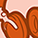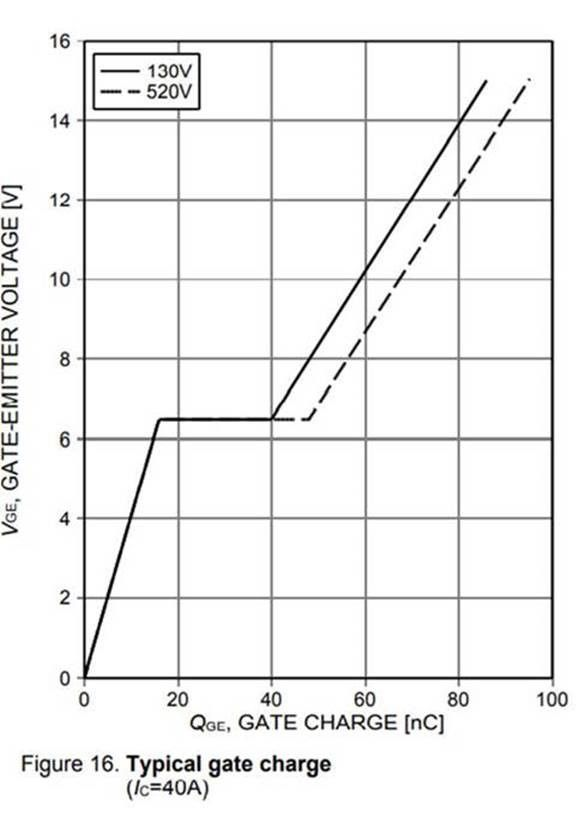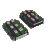# IGBT Forum DiscussionsLevel 1Level 1

# V_GE vs. Q_GE curve

Hi,

I have a question regarding V_GE vs. Q_GE curve of an IGBT. According to my understaning Q_GE is the charge stored in the gate-emitter capacitance(C_GE).
Now assume, that the gate driver is sourcing a constant current which will dump charges on C_GE and C_GC, thereof increasing the gate-emitter volatge till the platue region comes. At the onset of platue, the gate clamps its voltage and the source current (from the gate driver) will discharge the gate-collector capacitance (C_GC), which essentially means that during the platue region, no charges are being stored in C_GE, which in turn means in the ‘’V_GE vs. Q_GE’’ curve no flat region should be observed. However, V_GE vs. Q_GE curve given in the datasheet (picture attached), for Q_GE between (approx.) 15nC-40nC the gate-emitter voltage remains constant. So my question is, why the stored charge is increasing and not the voltage, given that C_GE remains constant?

Thank you1 SolutionModeratorModerator

# Re: V_GE vs. Q_GE curve

Hi JoFoe,
as you are writing correctly, the gate driver is sourcing a current which is dumping charges on C_GE and C_GC. So Q_GE is not only the charge stored in the gate-emitter capacitance(C_GE) but also the charge supplied to the gate-collector capacitance ( Miller capacitance C_GC).
Once the V_GE passes the V_GEth level, the IGBT turn-on process starts. V_CE starts dropping rapidly with high dv_CE/dt. While the V_CE is decreasing, the Miller capacitance C_GC is increasing. This is due to the fact, that the space charge region in the IGBT is spreading and by this increases the C_GC. The gate charge is flowing into the increasing capacitance, the gate voltage itself stays on the so called Miller plateau.
So your assumption regarding a constant C_GE in your question “why the stored charge is increasing and not the voltage, given that C_GE remains constant?” is not wrong, but did not consider the Miller capacitance which is charged by the gate current I_G during the Miller phase.
Regards
IGBT Wizzard
TSModeratorModerator

# Re: V_GE vs. Q_GE curve